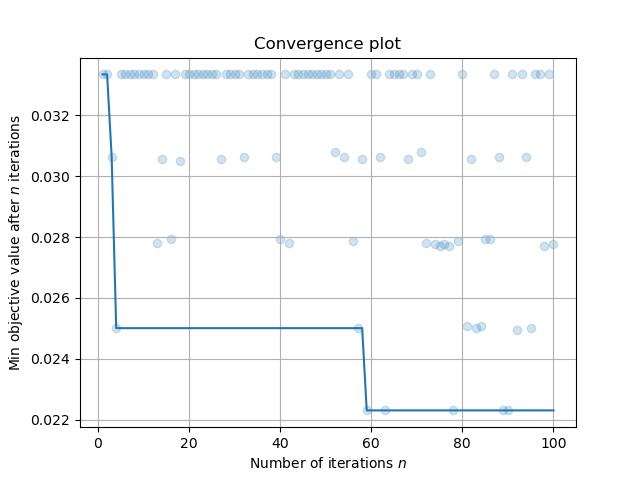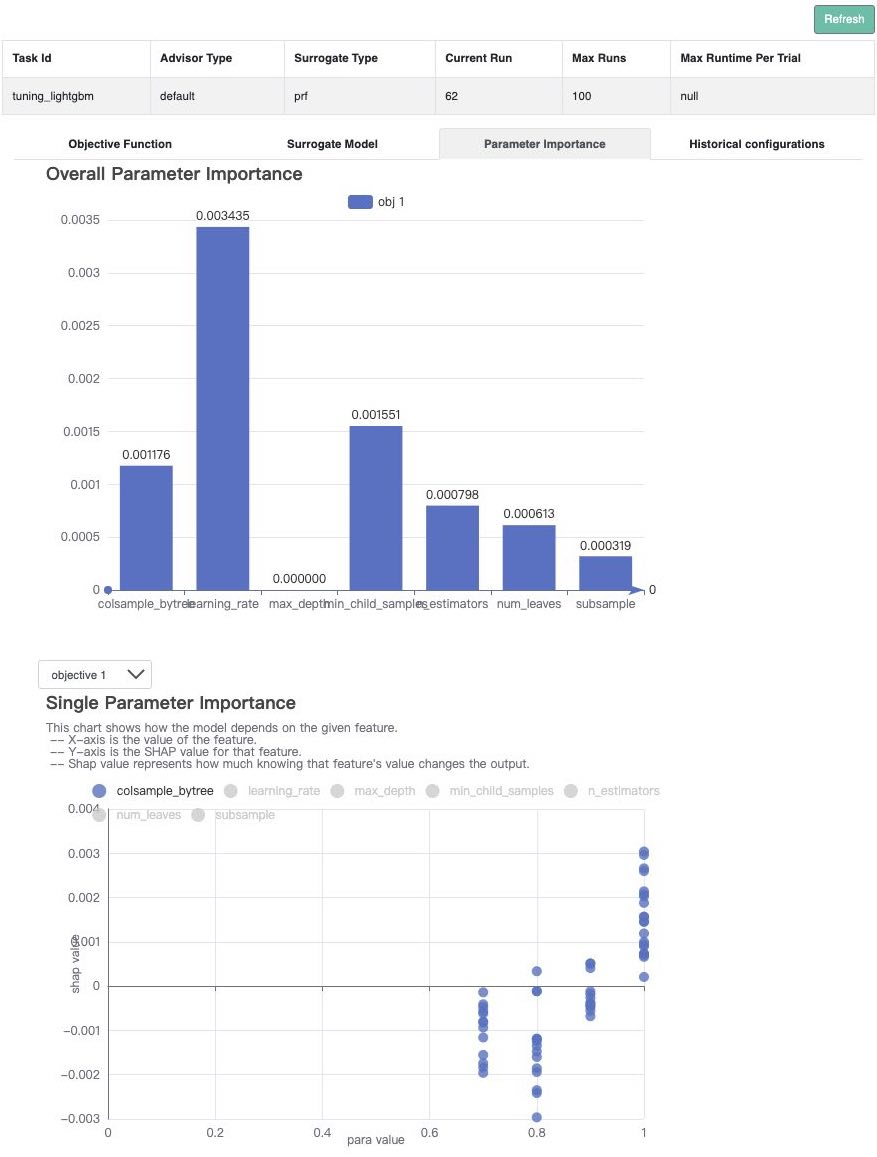# Single-Objective Black-box Optimization#

In this tutorial, we will introduce how to tune hyperparameters of ML tasks with OpenBox.

## Data Preparation#

First, prepare data for your ML model. Here we use the digits dataset from sklearn as an example.

# prepare your data
from sklearn.model_selection import train_test_split

x_train, x_test, y_train, y_test = train_test_split(X, y, test_size=0.2, stratify=y, random_state=1)


## Problem Setup#

Second, define the configuration space to search and the objective function to minimize. Here, we use LightGBM – a gradient boosting framework developed by Microsoft, as the classification model.

from openbox import space as sp
from sklearn.metrics import balanced_accuracy_score
from lightgbm import LGBMClassifier

def get_configspace():
space = sp.Space()
n_estimators = sp.Int("n_estimators", 100, 1000, default_value=500, q=50)
num_leaves = sp.Int("num_leaves", 31, 2047, default_value=128)
max_depth = sp.Constant('max_depth', 15)
learning_rate = sp.Real("learning_rate", 1e-3, 0.3, default_value=0.1, log=True)
min_child_samples = sp.Int("min_child_samples", 5, 30, default_value=20)
subsample = sp.Real("subsample", 0.7, 1, default_value=1, q=0.1)
colsample_bytree = sp.Real("colsample_bytree", 0.7, 1, default_value=1, q=0.1)
space.add_variables([n_estimators, num_leaves, max_depth, learning_rate, min_child_samples, subsample,
colsample_bytree])
return space

def objective_function(config: sp.Configuration):
params = config.get_dictionary().copy()
params['n_jobs'] = 2
params['random_state'] = 47

model = LGBMClassifier(**params)
model.fit(x_train, y_train)
y_pred = model.predict(x_test)

loss = 1 - balanced_accuracy_score(y_test, y_pred)  # minimize
return dict(objectives=[loss])


Here are some instructions on how to define a configuration space:

• When we define n_estimators, we set q=50, which means the values of the hyperparameter will be sampled at an interval of 50.

• When we define learning_rate, we set log=True, which means the values of the hyperparameter will be sampled on a logarithmic scale.

The input of the objective_function is a Configuration instance sampled from the space. You can call config.get_dictionary().copy() to convert Configuration into Python dict.

During this hyperparameter optimization task, once a new hyperparameter configuration is suggested, we rebuild the model based on the input configuration. Then, we fit the model, and evaluate the model’s predictive performance. These steps are carried out in the objective function.

After evaluation, the objective function returns a dict (Recommended). The result dictionary should contain:

• 'objectives': A list/tuple of objective values (to be minimized). In this example, we have only one objective so the tuple contains a single value.

• 'constraints': A list/tuple of constraint values. If the problem is not constrained, return None or do not include this key in the dictionary. Non-positive constraint values (“<=0”) imply feasibility.

In addition to returning a dictionary, for single-objective problems with no constraints, returning a single value is also supported.

## Optimization#

After defining the configuration space and the objective function, we can run the optimization process as follows:

from openbox import Optimizer

# Run
opt = Optimizer(
objective_function,
get_configspace(),
num_objectives=1,
num_constraints=0,
max_runs=100,
surrogate_type='prf',
# Have a try on the new HTML visualization feature!
# visualization='advanced',   # or 'basic'. For 'advanced', run 'pip install "openbox[extra]"' first
# auto_open_html=True,        # open the visualization page in your browser automatically
)
history = opt.run()


Here we create a Optimizer instance, and pass the objective function and the configuration space to it. The other parameters are:

• num_objectives=1 and num_constraints=0 indicate that our function returns a single value with no constraint.

• max_runs=100 means the optimization will take 100 rounds (optimizing the objective function 100 times).

• surrogate_type='prf'. For mathematical problem, we suggest using Gaussian Process ('gp') as Bayesian surrogate model. For practical problems such as hyperparameter optimization (HPO), we suggest using Random Forest ('prf').

• task_id is set to identify the optimization process.

• visualization: 'none', 'basic' or 'advanced'. See HTML Visualization.

• auto_open_html: whether to open the visualization page in your browser automatically. See HTML Visualization.

Then, opt.run() is called to start the optimization process.

## Visualization#

After the optimization, opt.run() returns the optimization history. Or you can call opt.get_history() to get the history. Then, call print(history) to see the result:

history = opt.get_history()
print(history)

+-------------------------+----------------------+
| Parameters              | Optimal Value        |
+-------------------------+----------------------+
| colsample_bytree        | 0.800000             |
| learning_rate           | 0.018402             |
| max_depth               | 15                   |
| min_child_samples       | 15                   |
| n_estimators            | 200                  |
| num_leaves              | 723                  |
| subsample               | 0.800000             |
+-------------------------+----------------------+
| Optimal Objective Value | 0.022305877305877297 |
+-------------------------+----------------------+
| Num Configs             | 100                  |
+-------------------------+----------------------+


Call history.plot_convergence() to visualize the optimization process:

import matplotlib.pyplot as plt
history.plot_convergence()
plt.show()Call print(history.get_importance()) to print the hyperparameter importance: (Note that you need to install the pyrfr package to use this function. Pyrfr Installation Guide

print(history.get_importance())

+-------------------+------------+
| Parameters        | Importance |
+-------------------+------------+
| learning_rate     | 0.293457   |
| min_child_samples | 0.101243   |
| n_estimators      | 0.076895   |
| num_leaves        | 0.069107   |
| colsample_bytree  | 0.051856   |
| subsample         | 0.010067   |
| max_depth         | 0.000000   |
+-------------------+------------+


In this task, the top-3 influential hyperparameters are learning_rate, min_child_samples, and n_estimators.

(New Feature!) Call history.visualize_html() to visualize the optimization process in an HTML page. For show_importance and verify_surrogate, run pip install "openbox[extra]" first. See HTML Visualization for more details.

history.visualize_html(open_html=True, show_importance=True,
verify_surrogate=True, optimizer=opt)